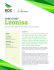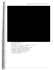The Z-Transform - Jingwei Zhu - Home

Transcription

The Z-Transform - Jingwei Zhu - Home
Jingwei Zhu
http://jingweizhu.weebly.com/course-note.html
The Z-Transform
The z-transform plays the same role for discrete-time signals and systems as does the Laplace transform
for continuous-time signals and systems.
The two-sided z-transform
The two-sided z-transform X(z) of a discrete signal x[n] is defined as
−
X(z) = ∑∞
(two-sided z-transform)
=−∞ []
The relation between x[n] and X(z) is denoted symbolically by
x[n] ⇔ ()
Here, x[n] and X(z) form a transform pair, and the double arrow implies a one-to-one correspondence
between the two.
The complex quantity z generalizes the concept of digital frequency F or Ω to the complex domain and is
described in polar form as
z = |r|ej2πF = || Ω
Values of z can be plotted on an Argand diagram called the z-plane.
(An Argand diagram is a plot of complex numbers as points z=x+iy in the complex plane using the x-axis
as the real axis and y-axis as the imaginary axis.)
The defining relation is a power series (Laurent series) in z. The term for each index k is the product of
the sample value x[k] and z −k.
For the sequence x[n]={-7,3,1⇐,4,-8,5}, the z-transform may be written as
X(z) = −7z −2 + 31 +  0 + 4 −1 − 8 −2 + 5 −3
Comparing x[n] and X(z), we observe that the quantity z −1 plays the role of a unit delay operator.
Since the defining relation for X(z) describes a power series, it may not converge for all z. The values of z
for which it does converge define the region of convergence (ROC) for X(z). Two completely different
sequences may produce the same two-sided z-transform X(z), but with different regions of convergence.
It is important (unlike Laplace transforms) that we specify the ROC associated with each X(z), especially
when dealing with the two-sided z-transform.
For example, the signal y[n] = −αn u[−n − 1], n = −1, −2, …. The two-sided z-transform of y[n], using
a change of variables, can be written as
1
Jingwei Zhu
http://jingweizhu.weebly.com/course-note.html
−1

= ∑ −( ) = −
=
, : || < ||

−
1
−
=1

∞
−
Y(z) = ∑ −
=−∞
The z-transform of αn [] is also z/(z − α), but with an ROC of |z| > |α|. We cannot uniquely identify a
signal from its transform alone unless we also specify the ROC.
The ROC of the z-transform X(z) determines the nature of the signal x[n]
Finite-length x[n]: ROC of X(z) is all the z-plane, except perhaps for z=0 and/or z = ∞.
Right-sided x[n]: ROC of X(z) is outside a circle whose radius is the largest pole magnitude.
Left-sided x[n]: ROC of X(z) is inside a circle whose radius is the smallest pole magnitude.
Two-sided x[n]: ROC of X(z) is an annulus bounded by the largest and smallest pole radius.
Poles, zeros, and the z-plane
The z-transform of many signals is a rational function of the form
X(z) =
N(z) B0 + 1  −1 + 2  −2 + ⋯ +   −
=
D(z)
1 + 1  −1 + 2  −2 + ⋯ +   −
Denote the roots of N(z) by zi , i=1,2,…,M and the roots of D(z) by pk , k=1,2,…,N, we may also express X(z)
in factored form as
X(z) =
K(z − z1 )(z − z2 ) … (z − zM )
(z − p1 )(z − p2 ) … (z − pN )
Assuming that common factors have been cancelled, the p roots of N(z) and the q roots of D(z) are
termed the zeros and the poles of the transfer function, respectively.
The transfer function
The response y[n] of a system with impulse response h[n], to an arbitrary input x[n], is given by the
convolution y[n] = x[n] ⋆ h[n]. Since the convolution operation transforms to a product, we have
Y(z) = X(z)H(z)  () = ()/()
(System response=the convolution of input and system impulse response. And convolution corresponds
to product in the z-domain. Therefore in z-domain, we can conveniently times the transfer function of
the system with the z-transformed input and achieve the z-transformed system response.)
Note that the transfer function is only defined for relaxed LTI systems, either as the ratio of the output
Y(z) and input X(z), or as the z-transform of the system impulse h[n].
A relaxed LTI system is also described by the difference equation:
2
Jingwei Zhu
http://jingweizhu.weebly.com/course-note.html
y[n] + A1 [ − 1] + ⋯ +  [ − ] = 0 [] + 1 [ − 1] + ⋯ +  [ − ]
Its z-transform results in the transfer function:
H(z) =
(z − z1 )(z − z2 ) … (z − zM )
Y(z) 0 + 1  −1 + ⋯ +   −
=
=K
−1
−
(z − p1 )(z − p2 ) … (z − pN )
X(z)
1 + 1  + ⋯ +
The poles of H(z) are called natural modes of natural frequencies. The poles of Y(z)=H(z)X(z) determine
the form of the system response. Clearly, the natural frequencies in H(z) will always appear in the
system response unless they are cancelled by any corresponding zeros in X(z). The zeros of H(z) may be
regarded as the (complex) frequencies that are blocked by the system. For causal systems, the number
of zeros cannot exceed the number of poles.
Stability of causal LTI systems
In the time domain, bounded-input bounded-output (BIBO) stability of an LTI system requires an
absolutely summable impulse response h[n].
Poles outside the unit circle (|z|>1) lead to exponential growth even if the input is bounded.
Multiple poles on the unit circle always result in polynomial growth
Simple (non-repeated) poles on the unit circle can also lead to an unbounded response.
None of these types of time-domain terms is absolutely summable, and their presence leads to system
instability.
If a system has simple (non-repeated) poles on the unit circle, it is sometimes called marginally stable.
If a system has all its poles and zeros inside the unit circle, it is called a minimum-phase system.
Stability of anti-causal systems
The stability of anti-causal systems requires that all the poles of H(z) lie outside and exclude the unit
circle.
The ROC of stable LTI systems always includes the unit cycle
The ROC of a stable system (be it causal, anti-causal, or two-sided) always includes the unit circle.
In the time domain, a causal system requires a causal impulse response h[n] with h[n]=0, n<0. In the zdomain, this is equivalent to a transfer function H(z) that is proper and whose ROC lies outside a circle of
finite radius. For stability, the poles of H(z) must lie inside the unit circle. Thus for a system to be both
causal and stable, the ROC must include the unit circle.
Similarly for a system to be both stable and anti-causal.
3

square, circle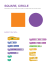2141005 - Gujarat Technological University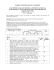PDF - Hotel Eden Roc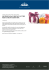fire stone home products outback chair company laneventure gloster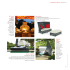Name: __________________________________________________________________ Period: ___________________youth newsletter september - White Mills Christian Church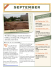Focus 40: Recovery is Breaking Through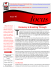Leonisa - ROC Commerce